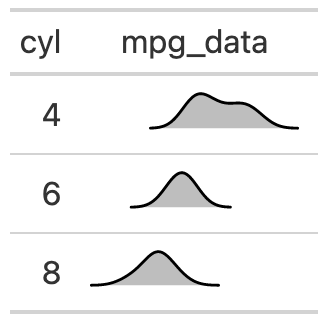The gt_plt_dist function takes an existing gt_tbl object and adds summary distribution sparklines via ggplot2. Note that these sparklines are limited to density, histogram, boxplot or rug/strip charts. If you're wanting to plot more traditional sparklines, you can use gtExtras::gt_plt_sparkline().

## Usage

gt_plt_dist(
gt_object,
column,
type = "density",
fig_dim = c(5, 30),
line_color = "black",
fill_color = "grey",
bw = NULL,
trim = FALSE,
same_limit = TRUE
)

## Arguments

gt_object

An existing gt table object of class gt_tbl

column

The column wherein the sparkline plot should replace existing data. Note that the data must be represented as a list of numeric values ahead of time.

type

A string indicating the type of plot to generate, accepts "boxplot", "histogram", "rug_strip" or "density".

fig_dim

A vector of two numbers indicating the height/width of the plot in mm at a DPI of 25.4, defaults to c(5,30)

line_color

Color for the line, defaults to "black". Accepts a named color (eg 'blue') or a hex color.

fill_color

Color for the fill of histograms/density plots, defaults to "grey". Accepts a named color (eg 'blue') or a hex color.

bw

The bandwidth or binwidth, passed to density() or ggplot2::geom_histogram(). If type = "density", then bw is passed to the bw argument, if type = "histogram", then bw is passed to the binwidth argument.

trim

A logical indicating whether to trim the values in type = "density" to a slight expansion beyond the observable range. Can help with long tails in density plots.

same_limit

A logical indicating that the plots will use the same axis range (TRUE) or have individual axis ranges (FALSE).

## Value

An object of class gt_tbl.

## Examples

 library(gt)
gt_sparkline_tab <- mtcars %>%
dplyr::group_by(cyl) %>%
# must end up with list of data for each row in the input dataframe
dplyr::summarize(mpg_data = list(mpg), .groups = "drop") %>%
gt() %>%
gt_plt_dist(mpg_data)

## Figures## Function ID

1-4

Other Plotting: gt_plt_bar_pct(), gt_plt_bar_stack(), gt_plt_bar(), gt_plt_percentile(), gt_plt_point(), gt_plt_sparkline(), gt_plt_winloss()